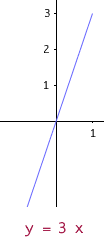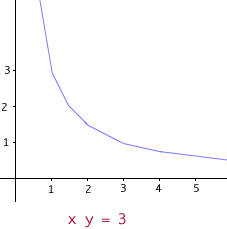Name: nancy ellis 12th grade physics student What is the difference between directly proportional and indirectly proportional? thanks! Hi Nancy, When two variables are directly proportional to each other, they are related by an equation such as the following: y = kx where k is a constant and x and y are variables. By examining this equation we see that when y is increased, it forces x to increase also since k is a constant and does not change. Similarly, when y is decreased, x also must decrease to maintain the equality. When a directly proportional relationship is graphed, the result is a linear graph with slope k and y-intercept at the origin.When two variables are indirectly proportional to each other (also known as inversely proportional), they are related by an equation of the following form: xy = k where k is a constant and x and y are variables. This equation may be re-written as y = k/x or x = k/y. It now becomes clearer to see that in an inversely proportional relationship, as one of the variables increases, the other decreases. The graph of an inversely proportional relationship displays a vertical asymptote at the y-axis as the y value increases without bound as x gets closer and closer to zero (but is positive) and decreases without bound as x gets closer and closer to zero (but is negative)and a horizontal asymptote at the x-axis as y gets closer and closer to zero as x increases or decreases without bound.Leeanne Go to Math Central